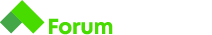# Practical Tasks - Arrays - Проблем с две от задачите

Здравейте, опитвам да реша две от задачите, но зациклих сериозно. Всякакви насоки са добре дошли. Поздрави!

# Signal from Space

One day a signal from space was registered. Scientists managed to record the signal as a sequence of `N` symbols. It turned out that due to a technical difficulty some symbols have been repeated. Help the scientists by writing a program which removes consecutive repetitions of symbols, decoding the message.

# Input

Input data is read from the standard input

• On the only input line, a message is given

# Output

Print to the standard output

• Print the decoded message

# Constraints

• The length of the message will be no more than `10000` symbols
• Message consists of digits and Latin letters (upper and lower case)

Hello

Helo

# Description

Ivancho considers a number to be “good”, if it can be divided by each of its digits.

For example:

• 13 is not “good”, because it cannot be divided by 3;
• 36 is “good”, because it can be divided both by 3 and 6;
• 102 is “good” - can be divided by 1 and by 2;
• 103 is not “good” - cannot be divided by 3;

Help Ivancho by writing a program, which counts the “good” numbers between number A and number B (inclusive).

# Input

Read from the standard input the numbers A and B received on one line, separated by space.

# Output

Print to the standard output the count of “good” numbers.

# Constraints

• 1 <= A <= B <= 100000

# Examples

1 10

#### Output

10

Explanation - between 1 and 10 there are 10 “good” numbers

42 142

29

256 768

#### Output

50

Здрасти, погледна ли този пост?

Здрасти, търсих доста, но тази тема съм я пропуснала.
Относно другата задача, мисля, че метода ми куца и накрая бройката не ми излиза, но не можах да си открия грешката.

Благодаря.

Здравей,
първо, counter-ът ти започва от 1.
А примерно при вход 57 58 , резултатът би трябвало да е 0.

после:

``````static boolean checkDivisibility (int n, int digit){
return (digit != 0 && n % digit == 0);
}
``````

Мисля, че ако числото = 0 , пак се брои, че се дели без остатък (поне според техния примерен инпут за 1 10, където резултатът е 10, т.е. всички числа са “добри”, дори 10)
т.е. май така ще трябва да стане:

`````` static boolean checkDivisibility(int n, int digit) {
return (digit == 0 || n % digit == 0);
}
``````

И последно тук:

``````
static boolean allDigitsDivide(int n) {
int temp = n;
while (temp > 0) {
int digit = n % 10; ----> тук n реално никога не се променя, то е извън цикъла. т.е. пробваш да делиш с едно и също число, докато намалиш достатъчно temp, за да излезе от while-a.
``````

вероятно си имала предвид да е int digit = temp % 10;

Btw, тук малко сложно го правиш:

``````            int firstNumber = 0;
int secondNumber = 0;

for (int i = 0; i < arr.length; i++) {
intArr[i] = Integer.parseInt(arr[i]);
if (i % 2 == 0){
firstNumber = intArr[i];
}
else{
secondNumber = intArr[i];
}
}
``````

може да го опростиш цялото до

``````        int firstNumber = Integer.parseInt(arr);
int secondNumber = Integer.parseInt(arr);
``````

УспехПроблема ми идва точно от проверката, ако числото е нула. Иначе си прав, за temp, това го видях, че не променя числото, но промених temp и пак не работеше, но сега всичко е ок.
Изключително благодаря и на двама Ви за насоките.

Здравейте,
Ето и моето решение на Good numbers. Нарочно съм се постарала да е по-различно от решенията до момента.

Здравейте и от мен,
до това решение достигнах аз. Дано е от помощ.
Поздрави!